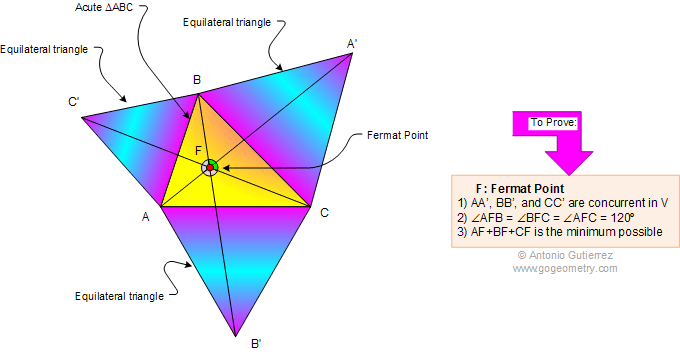Geometry Problem 903: First Fermat Point The figure shows an acute triangle ABC with three equilateral triangle erected externally on the sides. Prove that (1) AA', BB', and CC' concur in a point F called the Fermat point. (2)The angles AFB, BFC, and AFC are equal to 120 degrees. (3) AF + BF + CF is the minimum possible. The Fermat point of a triangle, is the solution to the problem of finding a point F such that the total distance from the three vertices of the triangle to the point is the minimum possible.Recent Additions < PREVIOUS PROBLEM  |  NEXT PROBLEM >